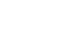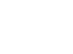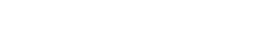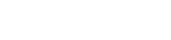## MATHEMATICS PAPER 2 - KCSE 2019 JOINT PRE MOCK EXAMINATION NAMBALE

SECTION I (50 MARKS)

Answer ALL the questions in this section.

1. Use logarithms to evaluate:(4 marks)1.
1. Expand (1- 1/2 x)6to fourth term.                                                                                   (2 marks)
2. Use the expansion above to evaluate (0.98)6 (2 marks)
1. The price of a new car is shs. 800,000. If it depreciates at a constant rate to shs. 550,000 within 4 years, find the annual rate of depreciation.  (3 marks)
1. Object A of the area 10cm2 is mapped onto its image B of area 60cm2 by a transformation whose matrix is given by. Find the positive values of x. (3 marks)
1. Without using a calculator or mathematical tables, express in surd form and simplify.          (3 marks)1. The position vector of A and B are a = 4i + 4j – 6k and b = 10i + 4j + 12 D is a point on AB such that AD:DB is 2:1. Find the co-ordinates of D. (3 marks)
1. A variable Z varies directly as the square of X and inversely as the square root of Y. Find percentage change in Z if X is increased by 20% and Y decreased by 19%. (3 marks)
1. Pipe A can fill a tank in 2 hours, Pipe B and C can empty the tank in 5 hours and 6 hours respectively. How long would it take:
1. To fill the tank if A and B are left open and C is closed. (2 marks)
2. To fill the tank with all pipes open. (2 marks)
1. Given that. Without using a mathematical table or a calculator, determine tan=(x+200)                      (3 marks)
1. Make P the subject of the formula XYP = QPX (3 marks)
1. The coordinates of the end points of diameter are A(2,4) B(-2,6). Find the equation of a circle in the form ax2 + by2 +cx + dy + e = 0  (3 marks)
1. A bag contains 10 balls of which 3 are red, 5 are white and 2 green. Another bag contains 12 balls of which 4 are red, 3 are white and 5 are green. A bag is chosen at random and a ball picked at random. Find the probability the ball so chosen is red. (3 marks)
1. The first, the second and sixth terms of an increasing arithmetic progression are the three consecutive terms of a geometric progression. If the first term of the arithmetic progression is 2, find:
1. Common difference of the arithmetic progression (2 marks)
2. Common ratio of the geometric progression. (1 mark)
1. Solve for x in the equation  (2 marks)2. The length and breadth of a rectangular floor garden were measured and found to be 4.1m and 2.2m respectively. Find the percentage error in its area.                                                                        (3 marks)
3. Given that 4y = 3 Sin 2/5x for 0≤x≤3600
1. Amplitude of the curve.                                                                                                 (1 mark)
2. Period of the curve. (2 marks)

SECTION II (50 MARKS)
Answer any FIVE questions in this section.

4. Asteel manufacturing factory had a sample of 5 iron rods of various lengths. The lengths of the rods were measured and recorded in the table below:
 Length (cm) 8-10 11-13 14-16 17-19 20-22 23-25 26-28 No. of rods 4 7 11 15 8 5 3

1. State the frequency of the modal class.             (1 mark)
2. Using 18 as an assumed mean, calculate:
1. Actual mean             (5 marks)
2. Variance             (3 marks)
3. Standard deviation                                                                                                                      (1 mark)
5. The above diagram represents a wooden prism. ABCD is a rectangle. Points E and F are directly below C and B respectively. M is the midpoint of CD. AB = 8cm, BC= 10cm and CE= 4.5cm.1. The size of angle CDE.             (2 marks)
2. Calculate:
1. Length of AC.                                                                                                                         (2 marks)
2. The angle CAE makes with the plane ADEF.             (2 marks)
3. Find the:
1. Length of MB.             (2 marks)
2. Angle CBM.             (2 marks)
6. Anaeroplane flies from a point P(600N, 450W) to a point Q(600N, 1350E). Given that the radius of the earth is 6370km,
1. Calculate the shortest distance between P and Q:
1. inkilometres (km)             (3 marks)
2. in nautical miles (nm)             (1 mark)
2. If the plane flew at a speed of 600 knots, how long did it take to move from P to Q.? (2 marks)
3. The plane left P at 10.00a.m on Monday. At what time did it arrive at Q if it travelled along a parallel latitude at the same speed.             (4 marks)
7. In the figure belowOA = p and OC = q. Vector AB = OC. Express in terms of unit vectors p and q the vectors.1.
1. AC                                                                                     (1 mark)
2. OB                                     (1 mark)
3. BC                                     (1 mark)
2. Vector AC intersects with vector OB at X such that AX = tAC and OX = hOB. By expressing OX in two ways in terms of t and h,
Find:
1. Scalars t and h             (5marks)
2. the ratio OB: BX             (2 marks)
8. Two fair dice one a regular tetrahedron (4 faces) and the other a cube are thrown. The scores are added together. Complete the table below to show all possible outcomes.  (2 marks)1. Find the probability that:
1. The sum is 6.             (1 mark)
2. The sum is an odd number.             (1 mark)
3. The sum is 6 or 9.                                                                                                                         (2 marks)
2. If a player wins a game by throwing a sum of 6 or 9, draw a tree diagram and use it to find probability that he wins at least once when the dice are thrown twice.                                      (4 marks)
9. The table below shows the income tax rates for a certain year.
 Taxable pay per month Ksh Tax rate 1 -9680 10% 9681 – 18800 15% 18801 – 27920 20% 27921 – 37040 25% 37040 – and above 30%

That year Mary paid net tax of Ksh.5,512   p.m. Her total monthly taxable allowances amounted to Ksh.15220 and he was entitled to a monthly relief of Ksh. 162. Every month the following deductions were made.
NHIF – Ksh.320
Union dues – Ksh.200
Co-operative shares – Ksh.7500
1. Calculate Mary’s monthly basic salary in Ksh.  (7 marks)
2. Calculate her monthly net salary.        (3 marks)
10. A number of people working at a factory decided to raise 72000 to buy a plot of land. Each person was to contribute the same amount. Before contributions five people retired from working at the factory and thus did not contribute. The same target of 72000 was still to be met by the remaining.
1. If n stands for the number of people working in the factory originally, show that the increase in the contribution per person was shs.360000/(n (n-5) (3 marks)
2. If the increase in contribution per person was sh.1200, find the number of people originally working at the factory. (4 marks)
3. Calculate the percentage increase in the contributions per person caused by retirement, giving your answer to one decimal place.  (3 marks)
11.
1. Complete the table below for the functions y = 3Sin x and y = 4Cos (2x - 10)                (2 marks)
 x 0 15 30 45 60 75 90 105 120 135 150 165 3Sin x 0 0.78 2.90 1.50 4Cos (2x-10) 3.94 2.57 3.06 -0.69 3.06
2. Using a scale of 1cm to rep 1 unit on the vertical axis and 1cm to rep 150 on the horizontal axis, draw both curves on the same axes.    (5 marks)
3. Use your curves to solve 3 Sin x – 4 Cos (2x -10) = 0 (2 marks)
4. State the phase angle of the curve y = 4 Cos (2x -10)                                                       (1 mark)## MARKING SCHEME############## Download MATHEMATICS PAPER 2 - KCSE 2019 JOINT PRE MOCK EXAMINATION NAMBALE.

• ✔ To read offline at any time.
• ✔ To Print at your convenience
• ✔ Share Easily with Friends / Students